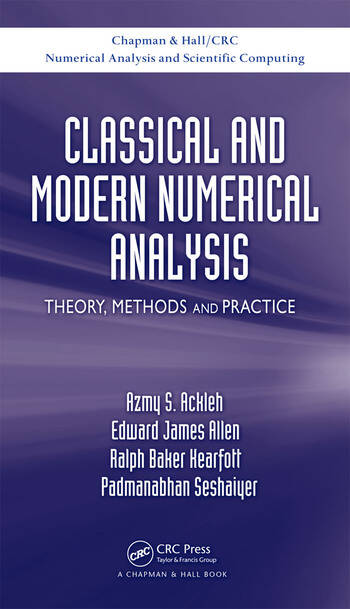# Classical and Modern Numerical Analysis: Theory, Methods and Practice

## 1st Edition

Azmy S. Ackleh, Edward James Allen, R. Baker Kearfott, Padmanabhan Seshaiyer

Chapman and Hall/CRC
Published July 20, 2009
Textbook - 628 Pages - 40 B/W Illustrations
ISBN 9781420091571 - CAT# C9157
Series: Chapman & Hall/CRC Numerical Analysis and Scientific Computing Series

For Instructors Request Inspection Copy

was \$150.00

USD\$120.00

SAVE ~\$30.00

FREE Standard Shipping!

## Preview

### Summary

Classical and Modern Numerical Analysis: Theory, Methods and Practice provides a sound foundation in numerical analysis for more specialized topics, such as finite element theory, advanced numerical linear algebra, and optimization. It prepares graduate students for taking doctoral examinations in numerical analysis.

The text covers the main areas of introductory numerical analysis, including the solution of nonlinear equations, numerical linear algebra, ordinary differential equations, approximation theory, numerical integration, and boundary value problems. Focusing on interval computing in numerical analysis, it explains interval arithmetic, interval computation, and interval algorithms. The authors illustrate the concepts with many examples as well as analytical and computational exercises at the end of each chapter.

This advanced, graduate-level introduction to the theory and methods of numerical analysis supplies the necessary background in numerical methods so that students can apply the techniques and understand the mathematical literature in this area. Although the book is independent of a specific computer program, MATLAB® code is available on the authors' website to illustrate various concepts.

#### Instructors

We provide complimentary e-inspection copies of primary textbooks to instructors considering our books for course adoption.